DCNet — Denoising (DNA) Sequence With a LSTM-RNN and PyTorch

Can we construct an error free consensus sequence from a set of noisy sequences using a neural network?

(associated Jupyter notebooks can be found at https://github.com/cschin/DCNet)

Using Neural Network to Remove Noise

Using a deep convolutional neural network (CNN) for denoising images or constructing super resolution images has generating some quite amazing results. The basic idea is that one can train a CNN to learn to remove the noisy pixels by training the network to construct the original image from those image corrupted by noises. Conceptually, one can think that the training process makes CNN to “learn” to do the best “guess” for replacing those missing/bad pixels in an corrupted image.

There is also another old technique, image stacking, to reduce image noises without any prior knowledge or a set of training images. The idea is to take many images of the same object, e.g., a dim planet or nebula in sky, and align multiple images together to reduce the noises. If the noisy pixels are generated randomly, then we can eliminate some pixels just appearing in one or two images as they are more likely to be random noises. The “correct” pixels of the object will likely be in all images. We can reconstruct a clearer image only use those pixels.

There is a good analogy of removing image noise to generating a better “consensus” (DNA) sequence from a set of noisy raw sequences generated by a DNA sequencer. The raw measurement from a DNA sequencer will have some errors. Depending on the underlying technologies, there might have different characteristics of the errors. One important bioinformatics task since the dawn of DNA sequencing is to remove errors and generate better consensus from repetitive measurements.

Unlike images, DNA sequences are one dimensional, discrete and represented by a small set of alphabets, A, C, G and T. This makes the denoising problem a bit easier if the errors are just “substitutional” (see my previous story). However, if there are errors from random insertion or deletion of the characters (= bases) in DNA sequences, then the problem is getting more complicated (for example, see the supplemental materials of the HGAP paper). Bioinformatics algorithm developers have developed a couple of methods for generating “consensus” sequences from a set of noisy sequences. Most of them uses some sort of pairwise sequence-sequence alignment or comparison for handling insertion and deletion errors. However, I am always intrigued about whether it is possible to generate a consensus sequence without any pairwise sequence-sequence alignment or comparison process.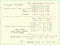Summary of generating consensus sequences from noisy measurment. If random substitution is the only error mode, it is trival to “stack” noisy sequences correctly, and it is easier to remove such random substitution errors. When there insert (extra character) errors and deletion (missing character) errors, how to stack the noisy sequences becomes a non-trivial problem. Both multiple sequence alignment and alignment graph are used to construct consensus in this case.

The Basic Idea

Can we “stack” a set of erroneous DNA sequences together to remove all kinds of errors with a neural network? If so, what should the network architecture look like? Maybe we can make a recurrent neural network (RNN), more specifically, an RNN with long short-term memory (LSTM) cells, to aggregate the correct sub-sequences and ignore some random erroneous events without doing explicitly alignments.

How do we make a LSTM-RNN to learn the correct parts and to remove errors without any prior knowledge about where the errors are? One idea we can borrow is from the architecture of an auto-encoder. In an auto-encoder, we typically try to learn some hidden structures of the data by pushing the data through a bottleneck layer and ask the second part of the network to reproduce the original data as the input of the network (check out the idea from this important paper: “Reducing the dimensionality of data with neural networks”). Such process forces the network to encode compressed information of the original data inside the neurons of the hidden layers.

One might argue the best compressed representation of a set of noisy sequences generated from an initial template sequence is just the initial template sequence. Motivated by the auto-encoder architecture, if we ask a LSTM-RNN to reproduce each noisy sequence we send into it, we might expect the network would learn the most common pattern likely from the error-free initial template sequence. If we ask it to generate a sequence from its “memory”, then it could just generate the correct template sequence from its “memory”.

A DNA Consensus LSTM Network (DCNet)

There are already many great tutorials online about the LSTM RNN. If you are not familiar with LSTM-RNN, I think colah's blog is one of the best places to get good background information about LSTM RNN. You will need it to understand how the consensus LSTM network perform its work. Personally, I won’t claim I understand LSTM-RNN fully but it is quite easy to implement a LSTM network with PyTorch. One can certainly learn some useful properties of a LSTM network by playing with it.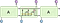The repeating module in an LSTM RNN contains four interacting layers. (image credit: colah’s blog)

One can simply consider that each of LSTM cell can learn a function mapping its inputs and the internal hidden states to some outputs. Once the network is trained, the hidden state may represent a particular sub-sequence that feed into the network before the particular LSTM cell. We can try to make a LSTM-RNN to predict a DNA characters given all previously known characters in the sequence. Namely, if we feed the first N DNA characters to the network, we want the Nth LSTM cell to predict the N+1'th character of the sequence correctly. In order to do this, we can set the loss function as the differences between the “predicted” DNA sequence output and the input sequence and train the network to minimize that.

This simple architecture of the DNA consensus LSTM network (DCNet) is shown below. We convert the input DNA sequences from a list of A,C,G, and T characters to a tensor consists of a list of one-hot encoded vectors of 4 elements of 0 or 1. We pass the output of each LSTM cell through two full connected ReLU layers and generate an output vector of four elements of float point numbers. We want the output vector to be as close as the representation of the next DNA character in the input sequence. Hence, we can define the loss function as the L1 or L2 loss between the predicted tensor and in the shifted input tensor.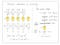The DCNet is a simple LSTM-RNN model. In the training, we make the LSTM cell to predict the next character (DNA base). We want to reduce the difference between the predicted sequence and the input sequence.

I implemented the DCNet with PyTorch. First, we generate some random sequence as the input template sequences. Then, we simulate 20 noisy sequences with insert, deletion and substitution errors and train the DCNet with only the noisy sequences.

Once the network is trained, we can test the DCNet to see if it can predict the original template sequence one step ahead correctly if we set the template as input. In my own experiments, sometimes the training process may not converge perfectly. One can adjust the training rate schedule or the mini-batch size to make the convergence more robust. It takes about 5 to 10 minutes using a NVIDIA GeForce GTX 1080 GPU.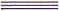The top plot represents the one-hot encoded matrix from the original template. The second one is the reconstruction from the the DCNet using the whole initial template as input. The third one is the difference between the two matrices. In some cases, if the training of the DCNet is not properly converged, you might see a lot of spot lighting up in the third matrix

Sending the template sequence to the DCNet to predict the sequence itself is not too useful. We like to see if we can construct the whole template sequence from the DCNet directly. To construct a sequence, we can just give a little hint to the DCNet by giving it the first character. After that, we can take the output of the Nth LSTM cell and send the output as the input to the N+1th LSTM cell after some small conversion to construct a sequence and compare it with the original template sequence to see how close they are to each other. (Note: thie DCNet framwork is very similar as predicting time series using neural network. If I will be looking to make this more efficient, I will search related papers in the time series analysis field.)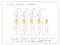When the DCNet is trained, we can just “prime” the network by giving the first character of the template sequences. Then, we iteratively use the previous output as the input for each LSTM to generate the consensus sequence.

Indeed, if the training converges well, we can reconstruct the original template sequences perfectly or with much fewer errors than those in the input noisy sequences used in the training. What have shown with this simple DCNet is that we can actually generate sequence consensus without doing any sequence-sequence comparison or alignment using a LSTM-RNN!!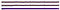Comparison of the generated consensus to the original template. If we have generate the consensus perfectly matching the original template, the elements of the third matrix will be all zeros (blue color).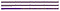The plots above show the consensus has some error around positon 70.

Some Interesting Extensions, Some Limitations and Some Random Thought

We have shown that a simple LSTM-RNN can generate short consensus sequence from noisy reads. While it is fun to construct a neural network to generate consensus sequences, the DCNet may not be a practical application as the speed is too slow than other solutions based on sequence alignment or comparison. However, there are some interesting properties of such network that is worth to think a bit more.

In the example shown above, each time when we train the network, we ask it to learn one consensus sequence. What if we send a couple different sets of noisy sequences from different templates and train the network, what will happen? Intuitively, if there is enough “memory capacity”, the DCNet may could learn multiple template sequences. As long as those template sequences are quite different from each other, so the sequence “paths” remembered by the DCNet don’t get mixed up.

Some quick experiment shows this is indeed the case when we use two totally independent generated sequences as the templates. Of course, one natural question to ask is about how many sequences can be reconstructed successful for such DCNet given a particular network architecture and hidden layer size. Also, we like to know how multiple template sequences affect training process? It is probably not easy to answer such theoretical questions without extensive research work.

In a lot of genomics work, one main challenge is to deal with some repetitive patterns in the DNA sequences. If there are repetitive patterns in the sequences, I think it will confuse the DCNet if the repetitive is too long and make it hard to generate correct consensus.

Can we use DCNet-like neural network for “homology” searching? Namely, identify (or fuzzy match) a known subsequence inside other much longer sequences.

In the canonical bioinformatics practices, such homology searching is done with so-call local sequence alignments or hidden Markov models(HMM). In the HMM approach, we need to specify a probabilistic model with certain structures of hidden states, transition and emission probabilities and use the results of multiple sequence alignments to train it.

In the DCNet, the iteration generating the next output from the previous output and the internal hidden state through each LSTM cell is just like the iteration step in a HMM. If we use the DCNet to scan through a long sequence and it starts to see some subsequences that it is “familiar” with, then the internal states likely go through some particular previous known trajectory too. We may be able to exploit such property and train another network using the output states and the internal states for finding homology sequences like using a HMM.

In contrast to the HMM approach like HMMER3, the DCNet model is arguably more generic. We don’t have to specify a particular probabilistic model which contain specific kinds of hidden states and emission probabilities. The network simply “learns” from the training data. This is analogous to by-passing the “feature engineering” phase in some machine learning tasks. Instead, we do need to work on “training engineering”. For example, if we like to use DCNet to find discontinuous homology sequences, we might need to have a training set with certain properties and adjust the training process to make sure the loss function is converging.

I have only tested the DCNet up to 200 characters of four alphabets. It does take a while for the training to converge. I don’t have a good idea on how long it will take to train the DCNet to work on much longer sequences. Alignment-based DNA consensus methods are way faster now and can handle sequences length up to hundred thousand or even millions of DNA bases. Is it possible to make the training much faster? If not, it may be still interesting to think whether there are other properties could make such network useful for some other applications. It is certainly easy to extend the alphabet sizes for sequence other than DNA. Or, instead of using one-hot input from DNA sequences, if we use word2vec embedding as input, can we catch some simple grammar errors or catch plagiarism? (I am sure there are already lots of work in the NLP field using more advanced network for this already.)

Written by